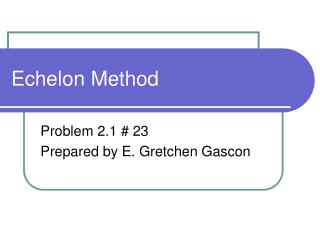# Echelon Method - PowerPoint PPT PresentationDownload PresentationEchelon Method

Echelon MethodDownload Presentation## Echelon Method

- - - - - - - - - - - - - - - - - - - - - - - - - - - E N D - - - - - - - - - - - - - - - - - - - - - - - - - - -
##### Presentation Transcript

1. Echelon Method Problem 2.1 # 23 Prepared by E. Gretchen Gascon

2. Step 1: The problem

3. Step 2: Eliminate the x term Use equation 1 and 2 Use the multiplication rule to get the coefficients of the x term opposites Next Add the two equations to get a new equation. Remember this equation it will be used later

4. Step 2: Eliminate the x term (cont.) Use equation 2 and 3 Use the multiplication rule to get the coefficients of the x term opposites Next Add the two equations to get a new equation. Remember this equation it will be used later

5. Step 3: Use the two equations to eliminate the y term Use the multiplication rule to get the coefficients of the y term opposites Next Add the two equations to get a new equation. You are now ready to create this new set of equations

6. The new system of equations One equations has 3 variables, one has 2 variables, and one equation has only 1 variable.

7. Step 6 Solve the last equation (the equation with only one variable)

8. Step 7: Back Substitution Start with the last equation (the simplest one)

9. There is more than one way to solve this problem The next slides will assume that you worked with r1 and r2, then when to use r1 and r3. The work looks a bit different, however the end results are the same.

10. (alternative solution) Step 2: Eliminate the x term (cont.) As demonstrated previous in step 2(slide #4) , you can use equation 2 and 3, however, you could have used equations 1 and 3 as well. This example uses 1 and 3 Multiply the r3 by -1 to get the coefficients as opposites Next Add the two equations to get a new equation. Notice that this equation can be solved for y already without further adding of equations .

11. Same Solutions